This tutorial demonstrates how to insert division signs in Excel.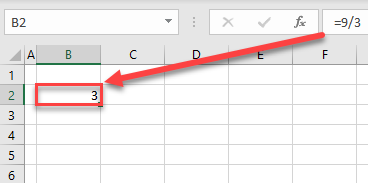## Divide Using Division Sign (/)

You can easily divide two numbers in Excel, using the division sign (/). Use it between the dividend and divisor in the cell:

``= dividend / divisor``

Say you want to divide 9 by 3 and get the result in cell B2. You need to enter in B2:

``=9/3``### Division – Cell References

Instead of constants, you can also divide using cell references. Say you have 10 in cell E2 and 4 in E3 and you want to divide them and get the result in cell B2. Type in the formula:

``=E2/E3``

in cell B2, as the dividend is in E2 and divisor is in E3.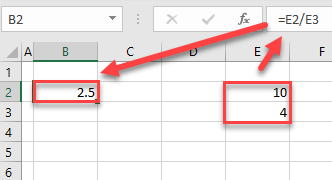The result is 2.5 in cell B2. If you change the value(s) in E2 or E3, the result of division in B2 updates accordingly.

### Division by Zero Error

If you try to divide any number by zero, you get the division by zero error (#DIV/0!). For example, entering:

``=10/0``

in cell B2 yields the division by zero error, shown below.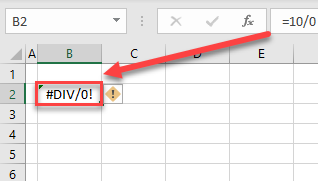If necessary, use the IF Function to avoid getting the #DIV/0! error.

## Insert Division Sign (÷) as Text

You can also insert a division sign (÷) as text, using symbols. Of course, this sign won’t calculate anything and is only read as text. This can be useful to visually explain the division calculation in an adjacent cell. For example, let’s say that in cell C2, you have the formula:

``=9/3``

and the result (3) is displayed. Now you want to make it clear how you got to this value; in cell B2, you want to enter 9 ÷ 3 =.

1. Type 9 and SPACE.
2. Then in the Ribbon, go to the Insert tab.
3. Choose Symbol.
4. Click the division sign (÷).
5. Click Insert.
6. After the symbol is inserted, complete the text with “ 3 =“.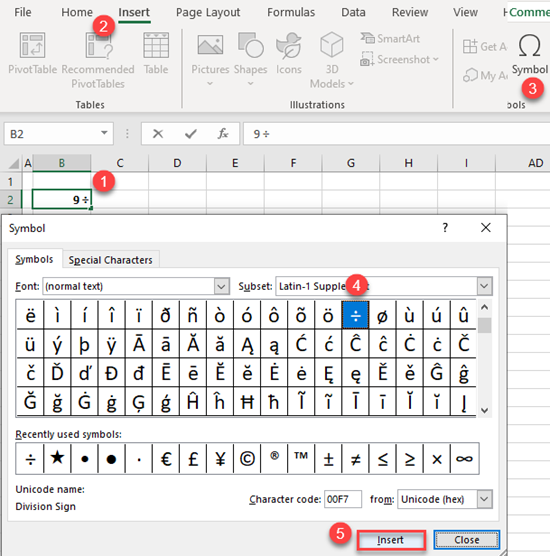Now the formula in C2 is visually described by the text in B2.

## Division Signs in Google Sheets

Everything mentioned for dividing using the division sign (/) in Excel works exactly the same in Google Sheets.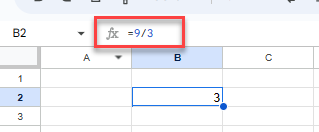Google Sheets does not have a symbols feature, so if you want to insert the text division sign (÷), you need to open Google Docs, and go to Insert > Special characters > Symbol > Math > Division Sign. Then copy and paste the symbol from the document into your worksheet.